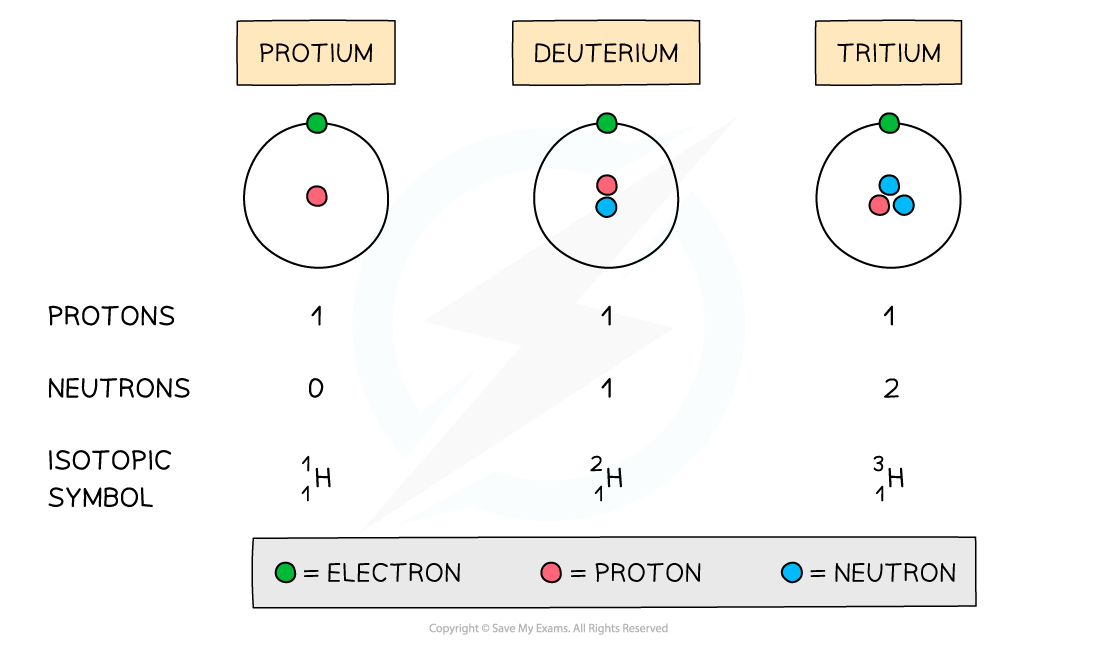# IB DP Chemistry: SL复习笔记2.1.2 Deducing Subatomic Particles

### Atoms: Key Terms

• The atomic number (or proton number) is the number of protons in the nucleus of an atom and has the symbol Z
• The atomic number is also equal to the number of electrons that are present in a neutral atom of an element
• E.g. the atomic number of lithium is 3, meaning that a neutral lithium atom has 3 protons and, therefore, also has 3 electrons
• The mass number (or nucleon number) is the total number of protons + neutrons in the nucleus of an atom, and has the  symbol A
• The number of neutrons can be calculated by:

Number of neutrons = mass number - atomic number

• Protons and neutrons are also called nucleons, because they are found in the nucleus

#### Exam TipThe mass (nucleon) and atomic (proton) number are given for each element in the Periodic Table

### Isotopes: Basics

• Isotopes are atoms of the same element that contain the same number of protons and electrons but a different number of neutrons
• The way to represent an isotope is to write the chemical symbol (or the word) followed by a dash and then the mass number
• E.g. carbon-12 and carbon-14 are isotopes of carbon containing 6 and 8 neutrons respectively
• These isotopes could also be written as 12C or C-12, and 14C or C-14 respectivelyThe atomic structure and symbols of the three isotopes of hydrogen

### Determining the Subatomic Structure of Atoms & Ions

• An atom is neutral and has no overall charge
• Ions on the other hand have either gained or lost electrons causing them to become charged
• The number of subatomic particles in atoms and ions can be determined given their atomic (proton) number, mass (nucleon) number and charge

#### Protons

• The atomic number of an atom and ion determines which element it is
• Therefore, all atoms and ions of the same element have the same number of protons (atomic number) in the nucleus
• E.g. lithium has an atomic number of 3 (three protons) whereas beryllium has atomic number of 4 (4 protons)
• The number of protons equals the atomic (proton) number
• The number of protons of an unknown element can be calculated by using its mass number and number of neutrons:

Mass number = number of protons + number of neutrons

Number of protons = mass number - number of neutrons

#### Worked Example

Determine the number of protons of the following ions and atoms:

1. Mg2+ ion
2. Carbon atom
3. An unknown atom of element X with mass number 63 and 34 neutrons

Answer 1: The atomic number of a magnesium atom is 12 suggesting that the number of protons in the magnesium element is 12

• Therefore the number of protons in a Mg2+ ion is also 12 - the number of protons does not change when an ion is formed

Answer 2: The atomic number of a carbon atom is 6 suggesting that a carbon atom has 6 protons in its nucleus

Answer 3: Use the formula to calculate the number of protons

Number of protons = mass number - number of neutrons

Number of protons = 63 - 34

Number of protons = 29

• Element X is therefore copper

#### Electrons

• An atom is neutral and therefore has the same number of protons and electrons
• Ions have a different number of electrons to the number of protons, depending on their charge
• A positively charged ion has lost electrons and therefore has fewer electrons than protons
• A negatively charged ion has gained electrons and therefore has more electrons than protons

#### Worked Example

Determine the number of electrons of the following ions and atoms:

1. Mg2+ ion
2. Carbon atom
3. An unknown atom of element X with mass number 63 and 34 neutrons

Answer 1: The atomic number of a magnesium atom is 12 suggesting that the number of protons in the neutral magnesium atom is 12

• However, the 2+ charge in Mg2+ ion suggests it has lost two electrons
• It only has 10 electrons left now

Answer 2: The atomic number of a carbon atom is 6 suggesting that the neutral carbon atom has 6 electrons orbiting around the nucleus

Answer 3: The number of protons of element X can be calculated by:

Number of protons = mass number - number of neutrons

Number of protons = 63 - 34

Number of protons = 29

• The neutral atom of element X  therefore also has 29 electrons

#### Neutrons

• The mass and atomic numbers can be used to find the number of neutrons in ions and atoms:

Number of neutrons = mass number (A) - number of protons (Z)

#### Worked Example

Determine the number of neutrons of the following ions and atoms:

1. Mg2+ ion
2. Carbon atom
3. An unknown atom of element X with mass number 63 and 29 protons

Answer 1: The atomic number of a magnesium atom is 12 and its mass number is 24

Number of neutrons = mass number (A) - number of protons (Z)

Number of neutrons = 24 - 12

Number of neutrons = 12

• The Mg2+ ion has 12 neutrons in its nucleus

Answer 2: The atomic number of a carbon atom is 6 and its mass number is 12

Number of neutrons = mass number (A) - number of protons (Z)

Number of neutrons = 12 - 6

Number of neutrons = 6

• The carbon atom has 6 neutrons in its nucleus

Answer 3: The atomic number of an element X atom is 29 and its mass number is 63

Number of neutrons = mass number (A) - number of protons (Z)

Number of neutrons = 63 - 29

Number of neutrons = 34

• The neutral atom of element X has 34 neutrons in its nucleus